## Mayday 'Fly to 2023' World Tour

We're proud to be the concert's official telco. Happening on 11th February 2023, win a pair of exclusive concert tickets to witness the legendary Chinese Rock band live in KL! #TTDoubleHappiness

#### Subscribe to any of the Fokus or Bebas plan to win MAYDAY Concert tickets!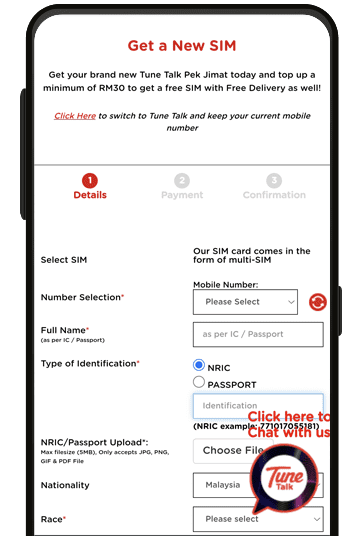STEP 1
Get a Pek Jimat from Tune Talk websiteSTEP 2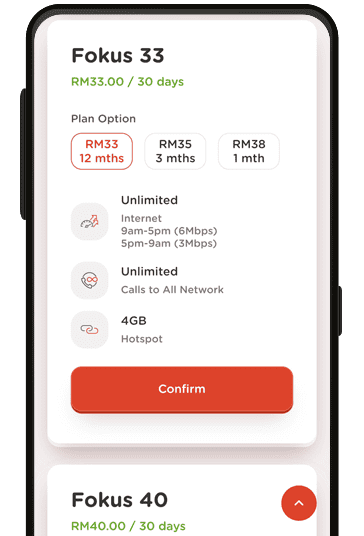STEP 3
Select your preferred Fokus or Bebas Plan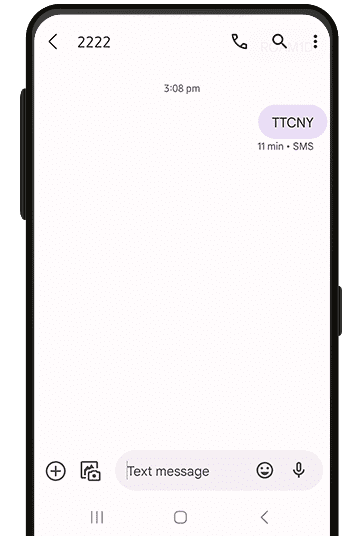STEP 4
SMS TTCNY to 2222 upon successful subscription.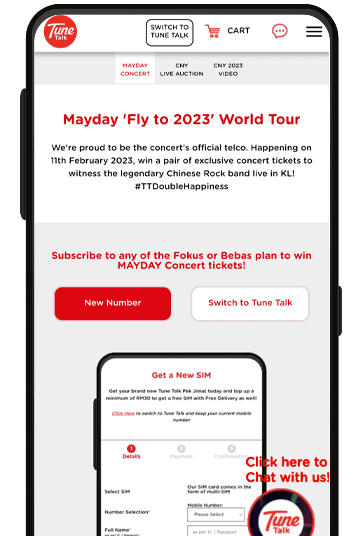STEP 5
Name of winners will be announced on Tune Talk social media platform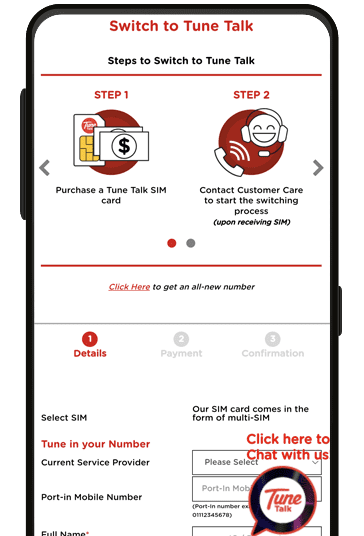STEP 1
Get a Pek Jimat from Tune Talk website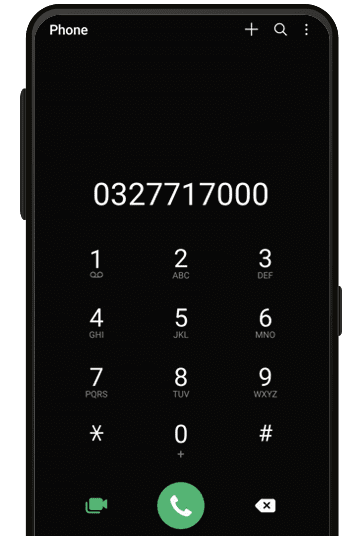STEP 2
Upon receiving the SIM card, contact our Customer Care to submit your switching request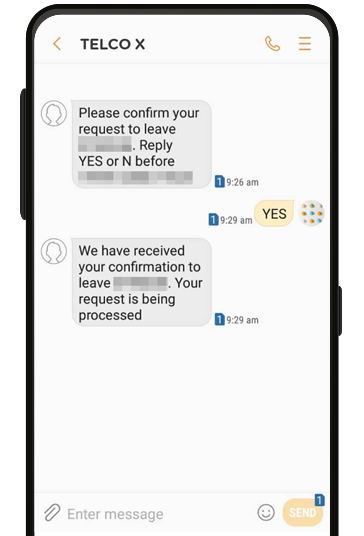STEP 3
*Switching process will take within 48 hours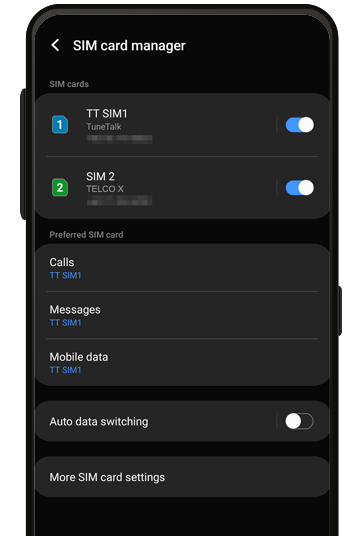STEP 4
Upon successful switch, swap your SIM cardSTEP 5STEP 6
Select your preferred Fokus or Bebas PlanSTEP 7
SMS TTCNY to 2222 upon successful subscription.STEP 8
Name of winners will be announced on Tune Talk social media platform

#### Win an iPad worth RM2,099!By subscribing to Pelan Fokus or Bebas, you're also in the running to win an iPad at our CNY Live Auction. A pop up message will appear in Tune Talk App for those who are eligible, kindly reply to Join

#### CNY Live Auction

Tune Talk FB Page | 10 February 2023 (8:30pm)#GoBeyond and get your Pek Jimat!

Top up a minimum of RM30 and we’ll deliver it to you for FREE!

from other telcos

with a new number

for existing subscribers

Response time: 1.0393009185791
1. select * from `rainlab_translate_attributes` where `locale` = ? and `model_id` = ? and `model_type` = ? limit 1
2. select * from `rainlab_translate_attributes` where `locale` = ? and `model_id` = ? and `model_type` = ? limit 1
3. select * from `rainlab_translate_attributes` where `locale` = ? and `model_id` = ? and `model_type` = ? limit 1
4. select * from `rainlab_translate_attributes` where `locale` = ? and `model_id` = ? and `model_type` = ? limit 1
5. select * from `rainlab_translate_attributes` where `locale` = ? and `model_id` = ? and `model_type` = ? limit 1
6. select * from `rainlab_translate_attributes` where `locale` = ? and `model_id` = ? and `model_type` = ? limit 1
7. select * from `rainlab_translate_attributes` where `locale` = ? and `model_id` = ? and `model_type` = ? limit 1
8. select * from `rainlab_translate_attributes` where `locale` = ? and `model_id` = ? and `model_type` = ? limit 1
9. select * from `rainlab_translate_attributes` where `locale` = ? and `model_id` = ? and `model_type` = ? limit 1
10. select * from `rainlab_translate_attributes` where `locale` = ? and `model_id` = ? and `model_type` = ? limit 1
11. select * from `rainlab_translate_attributes` where `locale` = ? and `model_id` = ? and `model_type` = ? limit 1
12. select * from `rainlab_translate_attributes` where `locale` = ? and `model_id` = ? and `model_type` = ? limit 1
13. select * from `rainlab_translate_attributes` where `locale` = ? and `model_id` = ? and `model_type` = ? limit 1
14. select * from `rainlab_translate_attributes` where `locale` = ? and `model_id` = ? and `model_type` = ? limit 1
15. select * from `rainlab_translate_attributes` where `locale` = ? and `model_id` = ? and `model_type` = ? limit 1
16. select * from `rainlab_translate_attributes` where `locale` = ? and `model_id` = ? and `model_type` = ? limit 1
17. select * from `rainlab_translate_attributes` where `locale` = ? and `model_id` = ? and `model_type` = ? limit 1
18. select * from `rainlab_translate_attributes` where `locale` = ? and `model_id` = ? and `model_type` = ? limit 1
19. select * from `rainlab_translate_attributes` where `locale` = ? and `model_id` = ? and `model_type` = ? limit 1
20. select * from `rainlab_translate_attributes` where `locale` = ? and `model_id` = ? and `model_type` = ? limit 1
21. select * from `rainlab_translate_attributes` where `locale` = ? and `model_id` = ? and `model_type` = ? limit 1
22. select * from `rainlab_translate_attributes` where `locale` = ? and `model_id` = ? and `model_type` = ? limit 1
23. select * from `rainlab_translate_attributes` where `locale` = ? and `model_id` = ? and `model_type` = ? limit 1
24. select * from `rainlab_translate_attributes` where `locale` = ? and `model_id` = ? and `model_type` = ? limit 1
25. select * from `rainlab_translate_attributes` where `locale` = ? and `model_id` = ? and `model_type` = ? limit 1
26. select * from `rainlab_translate_attributes` where `locale` = ? and `model_id` = ? and `model_type` = ? limit 1
27. select * from `rainlab_translate_attributes` where `locale` = ? and `model_id` = ? and `model_type` = ? limit 1
28. select * from `rainlab_translate_attributes` where `locale` = ? and `model_id` = ? and `model_type` = ? limit 1
29. select * from `rainlab_translate_attributes` where `locale` = ? and `model_id` = ? and `model_type` = ? limit 1
30. select * from `rainlab_translate_attributes` where `locale` = ? and `model_id` = ? and `model_type` = ? limit 1
31. select * from `rainlab_translate_attributes` where `locale` = ? and `model_id` = ? and `model_type` = ? limit 1
32. select * from `rainlab_translate_attributes` where `locale` = ? and `model_id` = ? and `model_type` = ? limit 1
33. select * from `rainlab_translate_attributes` where `locale` = ? and `model_id` = ? and `model_type` = ? limit 1
34. select * from `rainlab_translate_attributes` where `locale` = ? and `model_id` = ? and `model_type` = ? limit 1
35. select * from `rainlab_translate_attributes` where `locale` = ? and `model_id` = ? and `model_type` = ? limit 1
36. select * from `rainlab_translate_attributes` where `locale` = ? and `model_id` = ? and `model_type` = ? limit 1
37. select * from `rainlab_translate_attributes` where `locale` = ? and `model_id` = ? and `model_type` = ? limit 1
38. select * from `rainlab_translate_attributes` where `locale` = ? and `model_id` = ? and `model_type` = ? limit 1
39. select * from `rainlab_translate_attributes` where `locale` = ? and `model_id` = ? and `model_type` = ? limit 1
40. select * from `rainlab_translate_attributes` where `locale` = ? and `model_id` = ? and `model_type` = ? limit 1
41. select * from `rainlab_translate_attributes` where `locale` = ? and `model_id` = ? and `model_type` = ? limit 1
42. select * from `rainlab_translate_attributes` where `locale` = ? and `model_id` = ? and `model_type` = ? limit 1
43. select * from `rainlab_translate_attributes` where `locale` = ? and `model_id` = ? and `model_type` = ? limit 1
44. select * from `rainlab_translate_attributes` where `locale` = ? and `model_id` = ? and `model_type` = ? limit 1
45. select * from `rainlab_translate_attributes` where `locale` = ? and `model_id` = ? and `model_type` = ? limit 1
46. select * from `rainlab_translate_attributes` where `locale` = ? and `model_id` = ? and `model_type` = ? limit 1
47. select * from `rainlab_translate_attributes` where `locale` = ? and `model_id` = ? and `model_type` = ? limit 1
48. select * from `rainlab_translate_attributes` where `locale` = ? and `model_id` = ? and `model_type` = ? limit 1
49. select * from `rainlab_translate_attributes` where `locale` = ? and `model_id` = ? and `model_type` = ? limit 1
50. select * from `rainlab_translate_attributes` where `locale` = ? and `model_id` = ? and `model_type` = ? limit 1
51. select * from `rainlab_translate_attributes` where `locale` = ? and `model_id` = ? and `model_type` = ? limit 1
52. select * from `rainlab_translate_attributes` where `locale` = ? and `model_id` = ? and `model_type` = ? limit 1
53. select * from `rainlab_translate_attributes` where `locale` = ? and `model_id` = ? and `model_type` = ? limit 1
54. select * from `rainlab_translate_attributes` where `locale` = ? and `model_id` = ? and `model_type` = ? limit 1
55. select * from `rainlab_translate_attributes` where `locale` = ? and `model_id` = ? and `model_type` = ? limit 1
56. select * from `rainlab_translate_attributes` where `locale` = ? and `model_id` = ? and `model_type` = ? limit 1
57. select * from `rainlab_translate_attributes` where `locale` = ? and `model_id` = ? and `model_type` = ? limit 1
58. select * from `rainlab_translate_attributes` where `locale` = ? and `model_id` = ? and `model_type` = ? limit 1
59. select * from `rainlab_translate_attributes` where `locale` = ? and `model_id` = ? and `model_type` = ? limit 1
60. select * from `rainlab_translate_attributes` where `locale` = ? and `model_id` = ? and `model_type` = ? limit 1
61. select * from `rainlab_translate_attributes` where `locale` = ? and `model_id` = ? and `model_type` = ? limit 1
62. select * from `rainlab_translate_attributes` where `locale` = ? and `model_id` = ? and `model_type` = ? limit 1
63. select * from `rainlab_translate_attributes` where `locale` = ? and `model_id` = ? and `model_type` = ? limit 1
64. select * from `rainlab_translate_attributes` where `locale` = ? and `model_id` = ? and `model_type` = ? limit 1
65. select * from `rainlab_translate_attributes` where `locale` = ? and `model_id` = ? and `model_type` = ? limit 1
66. select * from `rainlab_translate_attributes` where `locale` = ? and `model_id` = ? and `model_type` = ? limit 1
67. select * from `rainlab_translate_attributes` where `locale` = ? and `model_id` = ? and `model_type` = ? limit 1
68. select * from `rainlab_translate_locales` where `code` = ? limit 1
69. select * from `tunetalk_front_page_blocks` where `tunetalk_front_page_blocks`.`parent_id` = ? and `tunetalk_front_page_blocks`.`parent_id` is not null
70. select `tunetalk_support_terms`.*, `tunetalk_front_contentable`.`content_id` as `pivot_content_id`, `tunetalk_front_contentable`.`contentable_id` as `pivot_contentable_id` from `tunetalk_support_terms` inner join `tunetalk_front_contentable` on `tunetalk_support_terms`.`id` = `tunetalk_front_contentable`.`contentable_id` where `tunetalk_front_contentable`.`content_id` = ? and `tunetalk_front_contentable`.`contentable_type` = ?
71. select * from `rainlab_translate_attributes` where `locale` = ? and `model_id` = ? and `model_type` = ? limit 1
72. select * from `rainlab_translate_attributes` where `locale` = ? and `model_id` = ? and `model_type` = ? limit 1
73. select * from `rainlab_translate_attributes` where `locale` = ? and `model_id` = ? and `model_type` = ? limit 1